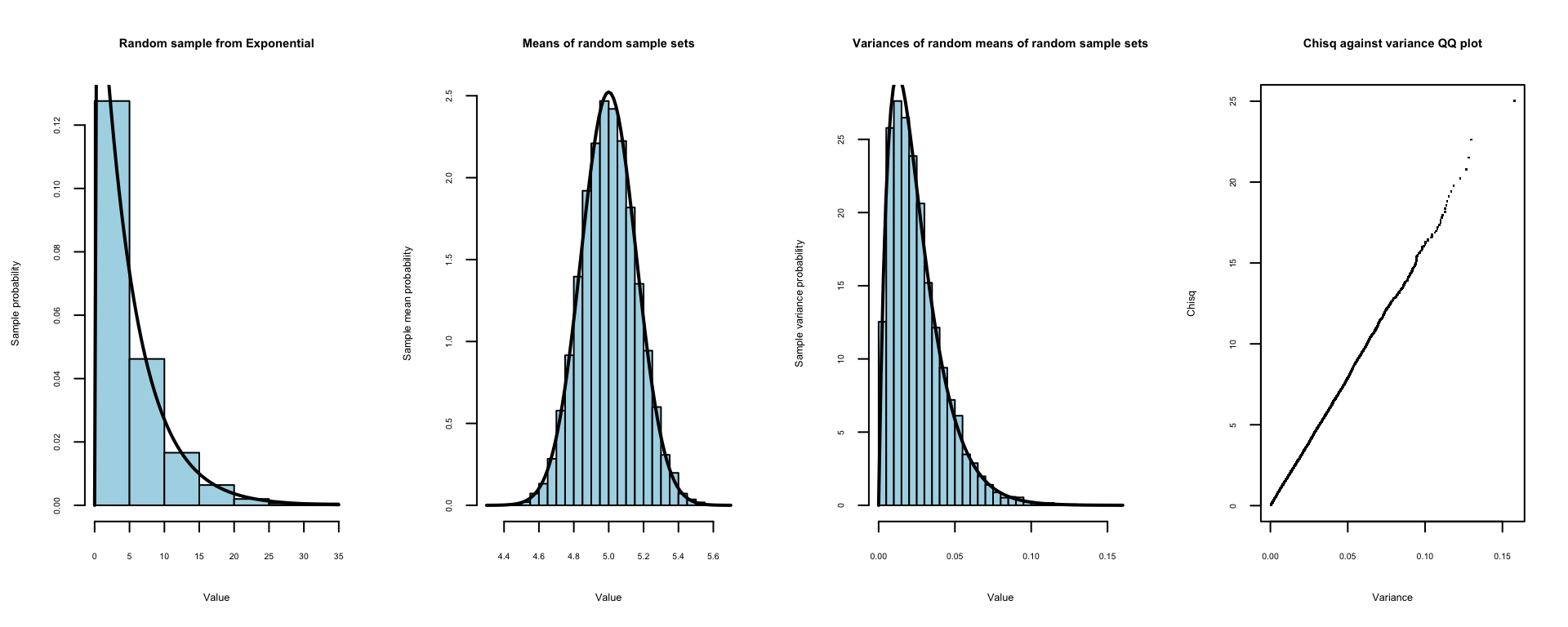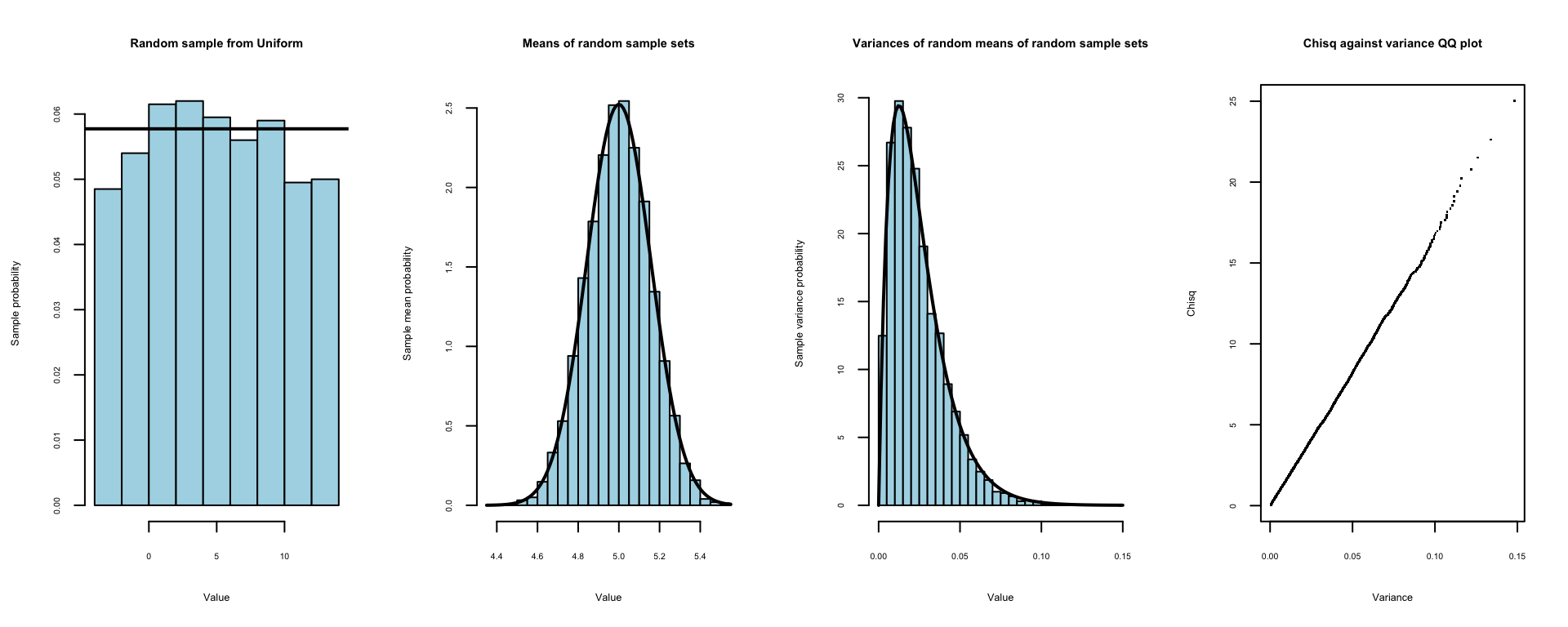This short paper presents the results of some simulations done to investigate the applicability of Cochran’s Theorem (CT) to a number of different probability distribution functions (pdfs). As is widely known, the Central Limit Theorem (CLT) states that, whatever the parent pdf, the distribution of the means of random samples from the parent pdf will tend to a normal distribution, provided the parent pdf is finite. What is less widely known is that CT states that the distribution of the variances of random samples of these normally distributed means will tend to a $$\chi^2$$ distribution with degrees of freedom = sample size of means - 1.

The pdfs initially selected for analysis were the exponential, uniform and normal pdfs as implemented in the R functions rexp(), runif() & rnorm(). This was later extended to cover the additional pdfs listed below. This work was carried out as an extension to the first part of the Data Science Specialization: Statistical Inference course project. The initial task of setting up the environment was carried out using the following code chunk.

## Make this reproducible by setting the random seed & other parameters
set.seed(1234)
pdf_var         <- 25
iterations      <- 10000
samplesize      <- 1000
mean_set_size   <- 5
xbreaks         <- 40
scaling         <- (mean_set_size-1)*(samplesize)/pdf_var
message ("Various parent distributions with variance = ", pdf_var,", sample size = ",
samplesize, " and iterations = ", iterations)
## Various parent distributions with variance = 25, sample size = 1000 and iterations = 10000
message ("Variances of means sample size = ", mean_set_size,", implies chisq dof = ", mean_set_size-1)
## Variances of means sample size = 5, implies chisq dof = 4

The next chunk sets up the function used to calculate and plot the distributions of the means of the random samples and the distributions of the variances of those means derived from a bootstrap procedure.

## Set-up required function
Distributions    <- function(mns, mean, sd, scaling, mean_set_size, iterations, xbreaks) {

## Plot means distribution
hist(mns, breaks = xbreaks, col = "lightblue", prob = TRUE,
main = "Means of random sample sets", xlab = "Value",
ylab = "Sample mean probability", cex.axis = 0.5, cex.lab = 0.6, cex.main = 0.7)
curve(dnorm(x, mean = mean, sd = sd), add = TRUE, yaxt = "n", lwd=2)

## Calculate and plot variances of means distribution histogram
vars        <- NULL
for (i in 1:(iterations)){vars    <- c(vars, var(sample(mns, mean_set_size, replace = TRUE)))}
hist(vars, breaks = xbreaks, col = "lightblue", prob = TRUE,
main = "Variances of random means of random sample sets", xlab = "Value",
ylab = "Sample variance probability", cex.axis = 0.5, cex.lab = 0.6, cex.main = 0.7)
curve(scaling*dchisq(x*scaling, df = (mean_set_size-1)), add = TRUE, yaxt = "n", lwd=2)

## Finally do a Q-Q plot for the variances against a chi-sqr distribution
qqplot(vars, qchisq(ppoints(vars), df = mean_set_size-1), pch=".", main = "Chisq against variance QQ plot",
xlab = "Variance", ylab = "Chisq", cex.axis = 0.5, cex.lab = 0.6, cex.main = 0.7)
return(NULL)
}

### Exponential pdf

The first pdf investigated was the exponential. The following code chunk was used to generate sets of random samples from an exponential distribution and evaluate the means of each of these sets and then the variances of sets of the means taken from the population of means via a bootstrap procedure. These results were then used to generate (i) a probability histogram showing an example of 1 set of random samples from the exponential pdf (with parent (exponential) pdf shown as a black line), (ii) the distribution of means from all sets of random samples (with normal pdf shown as a black line), (iii) the distribution of variances of sets of means bootstrapped from the population generated for (ii) (with $$\chi^2$$ pdf shown as a black line) and (iv) a QQ plot of the quantiles from a $$\chi^2$$ distribution against the quantiles from the variance data.

## This code block has been redacted since it contains the solution to one of the problems for the SI Project.The first histogram above shows an example random sample from the exponential distribution. The second histogram shows the compliance of the distribution of means with the CLT and the third histogram shows the compliance of the variance of the randomly sampled means with the CT. The fourth figure is a QQ plot showing the distribution of the quantiles from the $$\chi^2$$ distribution against the quantiles from the variance data. The linearity of the plot confirms the $$\chi^2$$ nature of the distribution of the variances.

The remainder of this report presents similar results to those above for examples of the following distributions:

• Uniform
• Normal
• Beta
• Binomial
• Cauchy
• Gamma
• Geometric
• Hypergeometric
• Logistic
• Log Normal
• Poisson’s
• Snedecor’s F
• Student’s t
• Weibull
• Wilcoxon Rank Sum Statistic
• Wilcoxon Signed Rank Statistic

### Uniform pdf

## Uniform pdf
par(mfrow = c(1, 4))

## Means of sample sets
meanUnif   <- sqrt(pdf_var)
minUnif    <- meanUnif - (sqrt(pdf_var*12)/2)
maxUnif    <- meanUnif + (sqrt(pdf_var*12)/2)
mns         <- NULL
sample      <- NULL
for (i in 1 : iterations) {
sample  <- runif(samplesize, minUnif, maxUnif)
mns     <- c(mns, mean(sample))
if (i==1) {
## Parent distribution histogram
hist(sample, col = "lightblue",
main = "Random sample from Uniform", xlab = "Value", prob = TRUE,
ylab = "Sample probability", cex.axis = 0.5, cex.lab = 0.6, cex.main = 0.7)
abline(h = (1/(maxUnif-minUnif)), lwd=2)
}
}

## Plot means distribution & variances of means distribution histograms
answer      <-   Distributions(mns, mean = meanUnif, sd = sqrt(pdf_var/samplesize), scaling,
mean_set_size, iterations, xbreaks)### Normal pdf

## Normal pdf
par(mfrow = c(1, 4))

## Means of sample sets
meanNorm   <- sqrt(pdf_var)
sdNorm     <- sqrt(pdf_var)
mns         <- NULL
sample      <- NULL
for (i in 1 : iterations) {
sample  <- rnorm(samplesize, meanNorm, sdNorm)
mns     <- c(mns, mean(sample))
if (i==1) {
## Parent distribution histogram
hist(sample, col = "lightblue",
main = "Random sample from Normal", xlab = "Value", prob = TRUE,
ylab = "Sample probability", cex.axis = 0.5, cex.lab = 0.6, cex.main = 0.7)
curve(dnorm(x, mean = meanNorm, sd = sdNorm), add = TRUE, yaxt = "n", lwd=2)
}
}

## Plot means distribution & variances of means distribution histograms
answer      <-   Distributions(mns, meanNorm, sd = (sdNorm/sqrt(samplesize)), scaling,
mean_set_size, iterations, xbreaks)